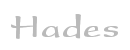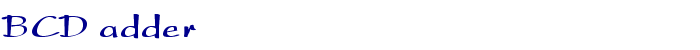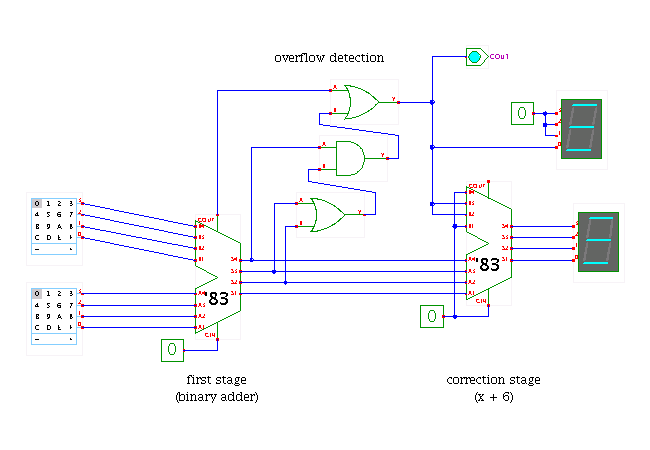TAMS / Java / Hades / applets (print version): contents | previous | next

#### Binary coded decimal adder (4 bit)Description

A binary coded decimal (BCD) adder. Note that you should only apply input values from 0..9 to the inputs of the adder, because the remaining values A..F are undefined for BCD arithmetic. Click the hex-switches or use the 'a' and 'b' bindkeys to select the input values for the adder.

Naturally, it would be easy to design a special circuit for the binary coded decimal arithmetic. However, this is seldom done.

The circuit shown here relies on the same trick that is often used in microprocessors for BCD arithmetic instructions. For example, many microprocessors including the Intel 808x and Motorola 68xx families provide a special decimal adjust accumulator instruction (DAA). A BCD addition is then performed in two steps, namely a standard addition followed by the DAA instruction. The basic operation performed by DAA is to add a constant value of 6 for each bcd-digit that overflowed during the first addition. Only very little logic is required to implement this operation.

To make this behaviour explicit, the circuit shown in the applet uses two stages of binary adders, each built with a single 7483 4-bit adder. The first stage consists of just the binary adder. The second stage uses a few gates to check for a decimal overflow, that is, output values larger than 9. If an overflow is detected, the second adder is hardwired to add the value 6 (0110) to the output of the first adder - which is equivalent to a subtraction of 10, thereby undoing the overflow of the first stage. The resulting 4-bit output value and 1-bit carry are the correct sum in BCD arithmetic.

Run the applet | Run the editor (via Webstart)

Impressum | 24.11.06